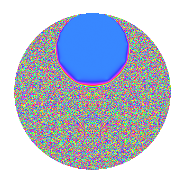# Properties

 Label 3.38.a.aLevel 3 Weight 38 Character orbit 3.a Self dual Yes Analytic conductor 26.014 Analytic rank 1 Dimension 3 CM No Inner twists 1

# Related objects

## Newspace parameters

 Level: $$N$$ = $$3$$ Weight: $$k$$ = $$38$$ Character orbit: $$[\chi]$$ = 3.a (trivial)

## Newform invariants

 Self dual: Yes Analytic conductor: $$26.0142114374$$ Analytic rank: $$1$$ Dimension: $$3$$ Coefficient field: $$\mathbb{Q}[x]/(x^{3} - \cdots)$$ Coefficient ring: $$\Z[a_1, \ldots, a_{5}]$$ Coefficient ring index: $$2^{9}\cdot 3^{5}\cdot 5$$ Fricke sign: $$1$$ Sato-Tate group: $\mathrm{SU}(2)$

## $q$-expansion

Coefficients of the $$q$$-expansion are expressed in terms of a basis $$1,\beta_1,\beta_2$$ for the coefficient ring described below. We also show the integral $$q$$-expansion of the trace form.

 $$f(q)$$ $$=$$ $$q + ( -103636 - \beta_{1} ) q^{2} -387420489 q^{3} + ( 112825533616 + 140608 \beta_{1} + 2 \beta_{2} ) q^{4} + ( -3209572628930 - 13500494 \beta_{1} - 39 \beta_{2} ) q^{5} + ( 40150709798004 + 387420489 \beta_{1} ) q^{6} + ( -1540628114567248 - 442709162 \beta_{1} - 35413 \beta_{2} ) q^{7} + ( -31127969697970496 - 95903083520 \beta_{1} - 621816 \beta_{2} ) q^{8} + 150094635296999121 q^{9} +O(q^{10})$$ $$q +(-103636 - \beta_{1}) q^{2} -387420489 q^{3} +(112825533616 + 140608 \beta_{1} + 2 \beta_{2}) q^{4} +(-3209572628930 - 13500494 \beta_{1} - 39 \beta_{2}) q^{5} +(40150709798004 + 387420489 \beta_{1}) q^{6} +(-1540628114567248 - 442709162 \beta_{1} - 35413 \beta_{2}) q^{7} +(-31127969697970496 - 95903083520 \beta_{1} - 621816 \beta_{2}) q^{8} +150094635296999121 q^{9} +(3566316712247265960 + 5957412808898 \beta_{1} + 33642688 \beta_{2}) q^{10} +(7557767785713209540 + 2973462571676 \beta_{1} + 34411854 \beta_{2}) q^{11} +(-43710923405196658224 - 54474420117312 \beta_{1} - 774840978 \beta_{2}) q^{12} +(-56090000067931697170 + 885146711869036 \beta_{1} - 657140938 \beta_{2}) q^{13} +($$$$26\!\cdots\!76$$$$+ 3598873140223312 \beta_{1} + 6916252224 \beta_{2}) q^{14} +($$$$12\!\cdots\!70$$$$+ 5230367987221566 \beta_{1} + 15109399071 \beta_{2}) q^{15} +($$$$10\!\cdots\!52$$$$+ 51201953589596160 \beta_{1} + 22823524896 \beta_{2}) q^{16} +(-$$$$90\!\cdots\!02$$$$+ 30058128433440564 \beta_{1} - 514120264806 \beta_{2}) q^{17} +(-$$$$15\!\cdots\!56$$$$- 150094635296999121 \beta_{1}) q^{18} +($$$$19\!\cdots\!68$$$$+ 434381446040702124 \beta_{1} + 5732394190422 \beta_{2}) q^{19} +(-$$$$13\!\cdots\!60$$$$- 3870883224028101248 \beta_{1} - 12284056198788 \beta_{2}) q^{20} +($$$$59\!\cdots\!72$$$$+ 171514600026820218 \beta_{1} + 13719721776957 \beta_{2}) q^{21} +(-$$$$14\!\cdots\!84$$$$- 9651854777049673412 \beta_{1} - 11807263879552 \beta_{2}) q^{22} +(-$$$$57\!\cdots\!24$$$$+ 8496445345062383212 \beta_{1} - 104980687284426 \beta_{2}) q^{23} +($$$$12\!\cdots\!44$$$$+ 37154819513926241280 \beta_{1} + 240904258788024 \beta_{2}) q^{24} +(-$$$$82\!\cdots\!25$$$$+$$$$13\!\cdots\!60$$$$\beta_{1} + 597373805525660 \beta_{2}) q^{25} +(-$$$$20\!\cdots\!48$$$$+ 61254426912333621778 \beta_{1} - 1658382321996672 \beta_{2}) q^{26} -$$$$58\!\cdots\!69$$$$q^{27} +(-$$$$67\!\cdots\!96$$$$-$$$$73\!\cdots\!76$$$$\beta_{1} - 3508458374889888 \beta_{2}) q^{28} +($$$$37\!\cdots\!26$$$$-$$$$82\!\cdots\!98$$$$\beta_{1} + 18257412042299043 \beta_{2}) q^{29} +(-$$$$13\!\cdots\!40$$$$-$$$$23\!\cdots\!22$$$$\beta_{1} - 13033866636234432 \beta_{2}) q^{30} +(-$$$$39\!\cdots\!12$$$$+$$$$13\!\cdots\!50$$$$\beta_{1} - 3843106330242889 \beta_{2}) q^{31} +(-$$$$90\!\cdots\!28$$$$-$$$$71\!\cdots\!32$$$$\beta_{1} - 20829013176835968 \beta_{2}) q^{32} +(-$$$$29\!\cdots\!60$$$$-$$$$11\!\cdots\!64$$$$\beta_{1} - 13331857304076606 \beta_{2}) q^{33} +(-$$$$62\!\cdots\!88$$$$+$$$$37\!\cdots\!94$$$$\beta_{1} + 27438424229580672 \beta_{2}) q^{34} +($$$$15\!\cdots\!60$$$$+$$$$53\!\cdots\!88$$$$\beta_{1} + 139088055761777178 \beta_{2}) q^{35} +($$$$16\!\cdots\!36$$$$+$$$$21\!\cdots\!68$$$$\beta_{1} + 300189270593998242 \beta_{2}) q^{36} +($$$$20\!\cdots\!22$$$$-$$$$73\!\cdots\!20$$$$\beta_{1} - 401057286573669456 \beta_{2}) q^{37} +(-$$$$12\!\cdots\!92$$$$-$$$$54\!\cdots\!96$$$$\beta_{1} - 1844989622710270848 \beta_{2}) q^{38} +($$$$21\!\cdots\!30$$$$-$$$$34\!\cdots\!04$$$$\beta_{1} + 254589863541878682 \beta_{2}) q^{39} +($$$$57\!\cdots\!00$$$$+$$$$13\!\cdots\!60$$$$\beta_{1} + 5209925388004786160 \beta_{2}) q^{40} +($$$$69\!\cdots\!78$$$$-$$$$44\!\cdots\!52$$$$\beta_{1} + 1156269906838666494 \beta_{2}) q^{41} +(-$$$$10\!\cdots\!64$$$$-$$$$13\!\cdots\!68$$$$\beta_{1} - 2679497818669417536 \beta_{2}) q^{42} +($$$$82\!\cdots\!48$$$$+$$$$33\!\cdots\!16$$$$\beta_{1} - 18160112109281379310 \beta_{2}) q^{43} +($$$$14\!\cdots\!24$$$$+$$$$21\!\cdots\!76$$$$\beta_{1} + 16584957391995795336 \beta_{2}) q^{44} +(-$$$$48\!\cdots\!30$$$$-$$$$20\!\cdots\!74$$$$\beta_{1} - 5853690776582965719 \beta_{2}) q^{45} +(-$$$$14\!\cdots\!52$$$$+$$$$11\!\cdots\!60$$$$\beta_{1} + 885320354412981376 \beta_{2}) q^{46} +(-$$$$39\!\cdots\!44$$$$-$$$$19\!\cdots\!56$$$$\beta_{1} + 49298541442184762322 \beta_{2}) q^{47} +(-$$$$41\!\cdots\!28$$$$-$$$$19\!\cdots\!40$$$$\beta_{1} - 8842301175911994144 \beta_{2}) q^{48} +(-$$$$74\!\cdots\!91$$$$+$$$$55\!\cdots\!72$$$$\beta_{1} + 39289783975049388704 \beta_{2}) q^{49} +(-$$$$32\!\cdots\!00$$$$-$$$$31\!\cdots\!95$$$$\beta_{1} -$$$$37\!\cdots\!20$$$$\beta_{2}) q^{50} +($$$$35\!\cdots\!78$$$$-$$$$11\!\cdots\!96$$$$\beta_{1} +$$$$19\!\cdots\!34$$$$\beta_{2}) q^{51} +($$$$14\!\cdots\!28$$$$+$$$$17\!\cdots\!40$$$$\beta_{1} +$$$$25\!\cdots\!80$$$$\beta_{2}) q^{52} +(-$$$$38\!\cdots\!94$$$$-$$$$17\!\cdots\!54$$$$\beta_{1} +$$$$11\!\cdots\!83$$$$\beta_{2}) q^{53} +($$$$60\!\cdots\!84$$$$+$$$$58\!\cdots\!69$$$$\beta_{1}) q^{54} +(-$$$$43\!\cdots\!60$$$$-$$$$14\!\cdots\!88$$$$\beta_{1} -$$$$44\!\cdots\!28$$$$\beta_{2}) q^{55} +($$$$21\!\cdots\!20$$$$+$$$$41\!\cdots\!04$$$$\beta_{1} +$$$$11\!\cdots\!24$$$$\beta_{2}) q^{56} +(-$$$$75\!\cdots\!52$$$$-$$$$16\!\cdots\!36$$$$\beta_{1} -$$$$22\!\cdots\!58$$$$\beta_{2}) q^{57} +($$$$19\!\cdots\!96$$$$-$$$$10\!\cdots\!70$$$$\beta_{1} -$$$$14\!\cdots\!04$$$$\beta_{2}) q^{58} +($$$$36\!\cdots\!12$$$$-$$$$73\!\cdots\!80$$$$\beta_{1} +$$$$19\!\cdots\!92$$$$\beta_{2}) q^{59} +($$$$52\!\cdots\!40$$$$+$$$$14\!\cdots\!72$$$$\beta_{1} +$$$$47\!\cdots\!32$$$$\beta_{2}) q^{60} +($$$$98\!\cdots\!34$$$$-$$$$26\!\cdots\!68$$$$\beta_{1} +$$$$71\!\cdots\!24$$$$\beta_{2}) q^{61} +($$$$82\!\cdots\!64$$$$+$$$$41\!\cdots\!12$$$$\beta_{1} -$$$$20\!\cdots\!00$$$$\beta_{2}) q^{62} +(-$$$$23\!\cdots\!08$$$$-$$$$66\!\cdots\!02$$$$\beta_{1} -$$$$53\!\cdots\!73$$$$\beta_{2}) q^{63} +(-$$$$35\!\cdots\!08$$$$+$$$$32\!\cdots\!12$$$$\beta_{1} +$$$$18\!\cdots\!52$$$$\beta_{2}) q^{64} +(-$$$$25\!\cdots\!20$$$$-$$$$27\!\cdots\!16$$$$\beta_{1} -$$$$22\!\cdots\!46$$$$\beta_{2}) q^{65} +($$$$57\!\cdots\!76$$$$+$$$$37\!\cdots\!68$$$$\beta_{1} +$$$$45\!\cdots\!28$$$$\beta_{2}) q^{66} +($$$$12\!\cdots\!48$$$$-$$$$19\!\cdots\!72$$$$\beta_{1} +$$$$51\!\cdots\!28$$$$\beta_{2}) q^{67} +(-$$$$71\!\cdots\!72$$$$-$$$$84\!\cdots\!88$$$$\beta_{1} -$$$$91\!\cdots\!56$$$$\beta_{2}) q^{68} +($$$$22\!\cdots\!36$$$$-$$$$32\!\cdots\!68$$$$\beta_{1} +$$$$40\!\cdots\!14$$$$\beta_{2}) q^{69} +(-$$$$14\!\cdots\!20$$$$-$$$$25\!\cdots\!96$$$$\beta_{1} -$$$$12\!\cdots\!76$$$$\beta_{2}) q^{70} +(-$$$$73\!\cdots\!48$$$$-$$$$97\!\cdots\!64$$$$\beta_{1} +$$$$10\!\cdots\!26$$$$\beta_{2}) q^{71} +(-$$$$46\!\cdots\!16$$$$-$$$$14\!\cdots\!20$$$$\beta_{1} -$$$$93\!\cdots\!36$$$$\beta_{2}) q^{72} +($$$$10\!\cdots\!26$$$$+$$$$70\!\cdots\!28$$$$\beta_{1} -$$$$11\!\cdots\!80$$$$\beta_{2}) q^{73} +($$$$15\!\cdots\!76$$$$+$$$$57\!\cdots\!18$$$$\beta_{1} +$$$$21\!\cdots\!40$$$$\beta_{2}) q^{74} +($$$$32\!\cdots\!25$$$$-$$$$53\!\cdots\!40$$$$\beta_{1} -$$$$23\!\cdots\!40$$$$\beta_{2}) q^{75} +($$$$11\!\cdots\!72$$$$+$$$$19\!\cdots\!76$$$$\beta_{1} +$$$$60\!\cdots\!08$$$$\beta_{2}) q^{76} +(-$$$$20\!\cdots\!56$$$$-$$$$17\!\cdots\!48$$$$\beta_{1} -$$$$26\!\cdots\!40$$$$\beta_{2}) q^{77} +($$$$79\!\cdots\!72$$$$-$$$$23\!\cdots\!42$$$$\beta_{1} +$$$$64\!\cdots\!08$$$$\beta_{2}) q^{78} +($$$$24\!\cdots\!80$$$$+$$$$70\!\cdots\!06$$$$\beta_{1} -$$$$62\!\cdots\!89$$$$\beta_{2}) q^{79} +(-$$$$20\!\cdots\!80$$$$-$$$$39\!\cdots\!64$$$$\beta_{1} -$$$$19\!\cdots\!84$$$$\beta_{2}) q^{80} +$$$$22\!\cdots\!41$$$$q^{81} +($$$$34\!\cdots\!04$$$$-$$$$74\!\cdots\!34$$$$\beta_{1} +$$$$69\!\cdots\!04$$$$\beta_{2}) q^{82} +(-$$$$10\!\cdots\!68$$$$+$$$$56\!\cdots\!92$$$$\beta_{1} +$$$$34\!\cdots\!70$$$$\beta_{2}) q^{83} +($$$$26\!\cdots\!44$$$$+$$$$28\!\cdots\!64$$$$\beta_{1} +$$$$13\!\cdots\!32$$$$\beta_{2}) q^{84} +($$$$70\!\cdots\!60$$$$+$$$$42\!\cdots\!88$$$$\beta_{1} +$$$$42\!\cdots\!78$$$$\beta_{2}) q^{85} +(-$$$$88\!\cdots\!20$$$$+$$$$95\!\cdots\!00$$$$\beta_{1} -$$$$35\!\cdots\!32$$$$\beta_{2}) q^{86} +(-$$$$14\!\cdots\!14$$$$+$$$$31\!\cdots\!22$$$$\beta_{1} -$$$$70\!\cdots\!27$$$$\beta_{2}) q^{87} +(-$$$$45\!\cdots\!76$$$$-$$$$11\!\cdots\!32$$$$\beta_{1} -$$$$54\!\cdots\!08$$$$\beta_{2}) q^{88} +(-$$$$53\!\cdots\!42$$$$+$$$$25\!\cdots\!52$$$$\beta_{1} +$$$$13\!\cdots\!60$$$$\beta_{2}) q^{89} +($$$$53\!\cdots\!60$$$$+$$$$89\!\cdots\!58$$$$\beta_{1} +$$$$50\!\cdots\!48$$$$\beta_{2}) q^{90} +($$$$15\!\cdots\!68$$$$-$$$$30\!\cdots\!04$$$$\beta_{1} -$$$$11\!\cdots\!98$$$$\beta_{2}) q^{91} +(-$$$$18\!\cdots\!08$$$$-$$$$20\!\cdots\!32$$$$\beta_{1} -$$$$86\!\cdots\!48$$$$\beta_{2}) q^{92} +($$$$15\!\cdots\!68$$$$-$$$$52\!\cdots\!50$$$$\beta_{1} +$$$$14\!\cdots\!21$$$$\beta_{2}) q^{93} +($$$$50\!\cdots\!00$$$$+$$$$18\!\cdots\!76$$$$\beta_{1} +$$$$30\!\cdots\!12$$$$\beta_{2}) q^{94} +(-$$$$35\!\cdots\!00$$$$-$$$$94\!\cdots\!80$$$$\beta_{1} -$$$$31\!\cdots\!80$$$$\beta_{2}) q^{95} +($$$$35\!\cdots\!92$$$$+$$$$27\!\cdots\!48$$$$\beta_{1} +$$$$80\!\cdots\!52$$$$\beta_{2}) q^{96} +($$$$89\!\cdots\!38$$$$-$$$$47\!\cdots\!52$$$$\beta_{1} -$$$$40\!\cdots\!52$$$$\beta_{2}) q^{97} +(-$$$$55\!\cdots\!00$$$$+$$$$50\!\cdots\!07$$$$\beta_{1} -$$$$17\!\cdots\!44$$$$\beta_{2}) q^{98} +($$$$11\!\cdots\!40$$$$+$$$$44\!\cdots\!96$$$$\beta_{1} +$$$$51\!\cdots\!34$$$$\beta_{2}) q^{99} +O(q^{100})$$ $$\operatorname{Tr}(f)(q)$$ $$=$$ $$3q - 310908q^{2} - 1162261467q^{3} + 338476600848q^{4} - 9628717886790q^{5} + 120452129394012q^{6} - 4621884343701744q^{7} - 93383909093911488q^{8} + 450283905890997363q^{9} + O(q^{10})$$ $$3q - 310908q^{2} - 1162261467q^{3} + 338476600848q^{4} - 9628717886790q^{5} + 120452129394012q^{6} - 4621884343701744q^{7} - 93383909093911488q^{8} + 450283905890997363q^{9} + 10698950136741797880q^{10} + 22673303357139628620q^{11} -$$$$13\!\cdots\!72$$$$q^{12} -$$$$16\!\cdots\!10$$$$q^{13} +$$$$79\!\cdots\!28$$$$q^{14} +$$$$37\!\cdots\!10$$$$q^{15} +$$$$32\!\cdots\!56$$$$q^{16} -$$$$27\!\cdots\!06$$$$q^{17} -$$$$46\!\cdots\!68$$$$q^{18} +$$$$58\!\cdots\!04$$$$q^{19} -$$$$40\!\cdots\!80$$$$q^{20} +$$$$17\!\cdots\!16$$$$q^{21} -$$$$44\!\cdots\!52$$$$q^{22} -$$$$17\!\cdots\!72$$$$q^{23} +$$$$36\!\cdots\!32$$$$q^{24} -$$$$24\!\cdots\!75$$$$q^{25} -$$$$61\!\cdots\!44$$$$q^{26} -$$$$17\!\cdots\!07$$$$q^{27} -$$$$20\!\cdots\!88$$$$q^{28} +$$$$11\!\cdots\!78$$$$q^{29} -$$$$41\!\cdots\!20$$$$q^{30} -$$$$11\!\cdots\!36$$$$q^{31} -$$$$27\!\cdots\!84$$$$q^{32} -$$$$87\!\cdots\!80$$$$q^{33} -$$$$18\!\cdots\!64$$$$q^{34} +$$$$47\!\cdots\!80$$$$q^{35} +$$$$50\!\cdots\!08$$$$q^{36} +$$$$60\!\cdots\!66$$$$q^{37} -$$$$37\!\cdots\!76$$$$q^{38} +$$$$65\!\cdots\!90$$$$q^{39} +$$$$17\!\cdots\!00$$$$q^{40} +$$$$20\!\cdots\!34$$$$q^{41} -$$$$30\!\cdots\!92$$$$q^{42} +$$$$24\!\cdots\!44$$$$q^{43} +$$$$42\!\cdots\!72$$$$q^{44} -$$$$14\!\cdots\!90$$$$q^{45} -$$$$43\!\cdots\!56$$$$q^{46} -$$$$11\!\cdots\!32$$$$q^{47} -$$$$12\!\cdots\!84$$$$q^{48} -$$$$22\!\cdots\!73$$$$q^{49} -$$$$96\!\cdots\!00$$$$q^{50} +$$$$10\!\cdots\!34$$$$q^{51} +$$$$43\!\cdots\!84$$$$q^{52} -$$$$11\!\cdots\!82$$$$q^{53} +$$$$18\!\cdots\!52$$$$q^{54} -$$$$12\!\cdots\!80$$$$q^{55} +$$$$63\!\cdots\!60$$$$q^{56} -$$$$22\!\cdots\!56$$$$q^{57} +$$$$58\!\cdots\!88$$$$q^{58} +$$$$10\!\cdots\!36$$$$q^{59} +$$$$15\!\cdots\!20$$$$q^{60} +$$$$29\!\cdots\!02$$$$q^{61} +$$$$24\!\cdots\!92$$$$q^{62} -$$$$69\!\cdots\!24$$$$q^{63} -$$$$10\!\cdots\!24$$$$q^{64} -$$$$75\!\cdots\!60$$$$q^{65} +$$$$17\!\cdots\!28$$$$q^{66} +$$$$38\!\cdots\!44$$$$q^{67} -$$$$21\!\cdots\!16$$$$q^{68} +$$$$66\!\cdots\!08$$$$q^{69} -$$$$43\!\cdots\!60$$$$q^{70} -$$$$22\!\cdots\!44$$$$q^{71} -$$$$14\!\cdots\!48$$$$q^{72} +$$$$32\!\cdots\!78$$$$q^{73} +$$$$46\!\cdots\!28$$$$q^{74} +$$$$96\!\cdots\!75$$$$q^{75} +$$$$34\!\cdots\!16$$$$q^{76} -$$$$61\!\cdots\!68$$$$q^{77} +$$$$23\!\cdots\!16$$$$q^{78} +$$$$74\!\cdots\!40$$$$q^{79} -$$$$61\!\cdots\!40$$$$q^{80} +$$$$67\!\cdots\!23$$$$q^{81} +$$$$10\!\cdots\!12$$$$q^{82} -$$$$31\!\cdots\!04$$$$q^{83} +$$$$78\!\cdots\!32$$$$q^{84} +$$$$21\!\cdots\!80$$$$q^{85} -$$$$26\!\cdots\!60$$$$q^{86} -$$$$43\!\cdots\!42$$$$q^{87} -$$$$13\!\cdots\!28$$$$q^{88} -$$$$16\!\cdots\!26$$$$q^{89} +$$$$16\!\cdots\!80$$$$q^{90} +$$$$45\!\cdots\!04$$$$q^{91} -$$$$54\!\cdots\!24$$$$q^{92} +$$$$45\!\cdots\!04$$$$q^{93} +$$$$15\!\cdots\!00$$$$q^{94} -$$$$10\!\cdots\!00$$$$q^{95} +$$$$10\!\cdots\!76$$$$q^{96} +$$$$26\!\cdots\!14$$$$q^{97} -$$$$16\!\cdots\!00$$$$q^{98} +$$$$34\!\cdots\!20$$$$q^{99} + O(q^{100})$$

Basis of coefficient ring in terms of a root $$\nu$$ of $$x^{3} - x^{2} - 2495042360 x + 9241471873200$$:

 $$\beta_{0}$$ $$=$$ $$1$$ $$\beta_{1}$$ $$=$$ $$12 \nu - 4$$ $$\beta_{2}$$ $$=$$ $$72 \nu^{2} + 399936 \nu - 119762166616$$
 $$1$$ $$=$$ $$\beta_0$$ $$\nu$$ $$=$$ $$($$$$\beta_{1} + 4$$$$)/12$$ $$\nu^{2}$$ $$=$$ $$($$$$\beta_{2} - 33328 \beta_{1} + 119762033304$$$$)/72$$

## Embeddings

For each embedding $$\iota_m$$ of the coefficient field, the values $$\iota_m(a_n)$$ are shown below.

For more information on an embedded modular form you can click on its label.

Label $$\iota_m(\nu)$$ $$a_{2}$$ $$a_{3}$$ $$a_{4}$$ $$a_{5}$$ $$a_{6}$$ $$a_{7}$$ $$a_{8}$$ $$a_{9}$$ $$a_{10}$$
1.1
 47984.3 3724.64 −51708.0
−679444. −3.87420e8 3.24205e11 −1.35264e13 2.63231e14 −4.10476e15 −1.26897e17 1.50095e17 9.19042e18
1.2 −148328. −3.87420e8 −1.15438e11 7.60742e11 5.74652e13 2.59260e15 3.75086e16 1.50095e17 −1.12839e17
1.3 516864. −3.87420e8 1.29709e11 3.13693e12 −2.00244e14 −3.10972e15 −3.99525e15 1.50095e17 1.62136e18
 $$n$$: e.g. 2-40 or 990-1000 Significant digits: Format: Complex embeddings Normalized embeddings Satake parameters Satake angles

## Inner twists

This newform does not admit any (nontrivial) inner twists.

## Atkin-Lehner signs

$$p$$ Sign
$$3$$ $$1$$

## Hecke kernels

This newform can be constructed as the kernel of the linear operator $$T_{2}^{3} + 310908 T_{2}^{2} - 327064838400 T_{2} -$$$$52\!\cdots\!24$$ acting on $$S_{38}^{\mathrm{new}}(\Gamma_0(3))$$.